#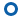Article
• Dependence of the Thermal Conductivity of PMMA, PS and PE on Temperature and Crystallinity
• B. Salameh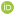, S. Yasin*, D. Abu Fara**, and A. M. Zihlif***

• Department of Applied Physics, Tafila Technical University, Tafila, Jordan
*College of Engineering and Technology, American University of the Middle East, Kuwait
**Chemical Engineering Department, the University of Jordan, Amman-Jordan
***Physics Department, the University of Jordan, Amman-Jordan

• 온도 및 결정화도에 따른 PMMA, PS 및 PE의 열전도도
• Reproduction, stored in a retrieval system, or transmitted in any form of any part of this publication is permitted only by written permission from the Polymer Society of Korea.

Abstract

This study presents measurements of the thermal conductivity as a function of temperature for different polymers: poly(methyl methacrylate) (PMMA), polystyrene (PS) and polyethylene (PE). The effects of varying the polymer structure, sample thickness and degree of crystallinity on the thermal conductivity are reported. The thermal conductivity increases with temperature for amorphous and semicrystalline polymers with crystallinity less than 50%, and decreases with temperature for semicrystalline polymers with crystallinity larger than 50%. Moreover, the thermal conductivity of the crystalline polymer is found to be larger than that of the amorphous polymer at the same temperature. The contact resistance of PMMA and PE could be calculated from the sample thickness dependence of the thermal resistance

The thermal conductivity as a function of temperature for three different polymers: polymethylmethacrylate, polystyrene and polyethylene was measured. The thermal conductivity increases with temperature for amorphous and semicrystalline polymers with crystallinity less than 50%, and decreases with temperature for semicrystalline polymers with crystallinity larger than 50%.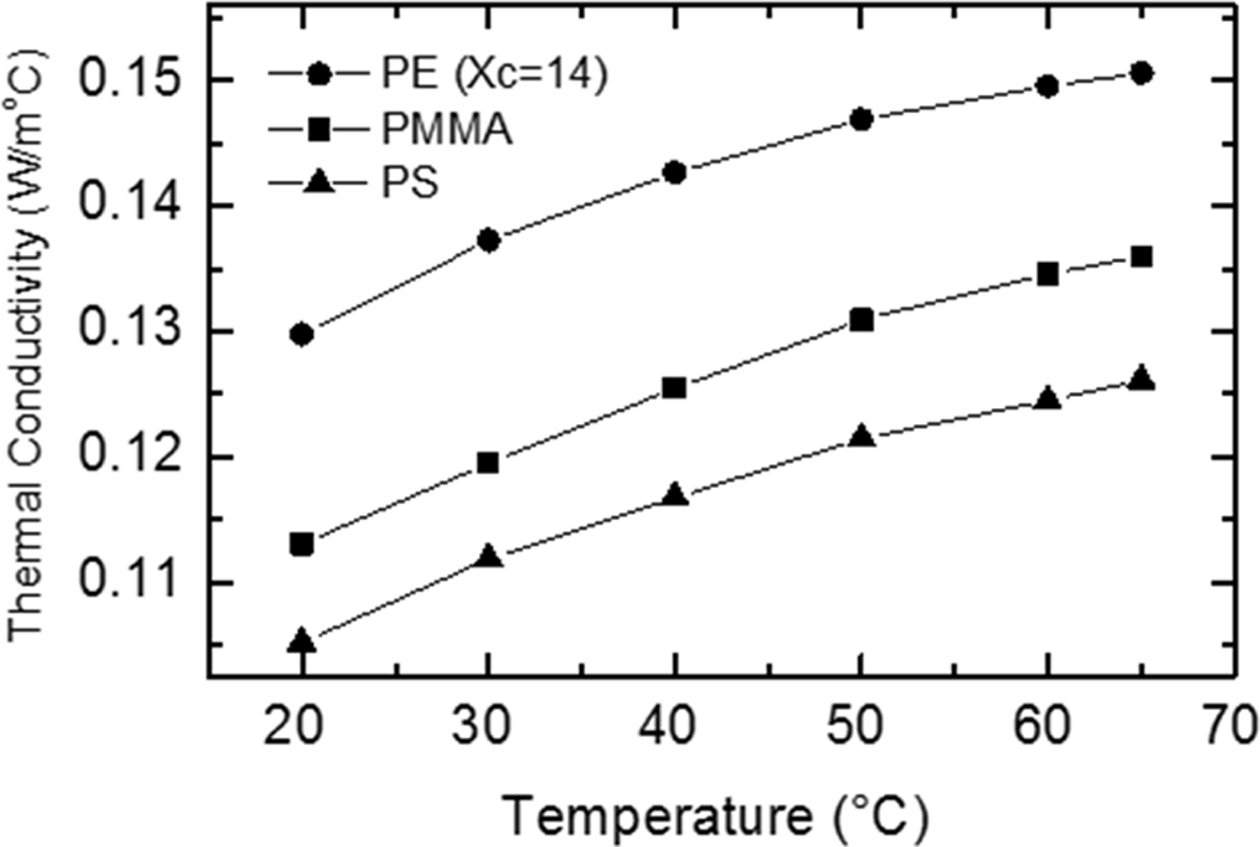Keywords: thermal conductivity, thermal diffusivity, semicrystalline polymers, crystallinity

Acknowledgements

The authors thank the University of Applied Science (Jordan), and the Jordanian Pharmaceutical Manufacturing Company for their cooperation

introduction

Nowadays, polymeric materials play a crucial role in our daily life. They are widely used in various fields, including aeronautics and astronautics devices, thermal insulators, integrated circuit, environmental protection and biomedical science.1-4 The importance of polymers has become much more emphasized as its applications are numerous and varied. Poly (methyl methacrylate) (PMMA), polystyrene (PS) and polyethylene (PE) have several applications in different fields including aerospace, automotive, electronics, packaging and medical applications.5 They have several desirable properties, including high strength, excellent dimensional stability, and exceptional optical clarity. These materials are widely used in adhesives, lens, neon signs, automotive signal light, paper coatings, etc. However, just like most polymers, they are thermal insulation materials, which limits their applications. In order to maximize their performance, polymers are increasingly required in situations that challenge the thresholds of their thermal capabilities. It is therefore important to study the thermal properties of these materials under a wide range of conditions relevant to industrial processing and use.
Heat conduction in polymers is mediated mainly by the elastic wave of phonons only as the result of the molecular vibration of the lattice, where there are no free electrons.6 Thermal conductivity of polymers can be described by Debye and Eiermann model7-10 as: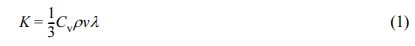where, K is the thermal conductivity, Cv is the heat capacity at constant volume, ρ is the density, v is the propagation speed of the phonons through the lattice (sound velocity) and λ is the mean free path of phonon. Under steady-state conditions when the temperature is constant, the thermal conductivity can be calculated using the relation10-12: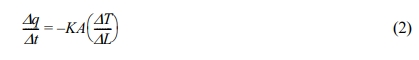where Δqt is the heat flow rate, A is the sample cross section area, ΔT is the temperature difference between the specimen faces, and ΔL is the specimen thickness. The thermal diffusivity D can be calculated from the thermal conductivity using the relation7: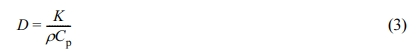where Cp is the specific heat at constant volume. To calculate the thermal diffusivity as a function of temperature we assume that the density is independent of temperature, and we calculate the specific heat as a function of temperature from the eq.12: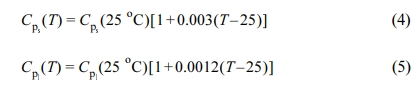The subscript s refers to the solid phase and l to the liquid phase. For PS, PMMA and PE (with 100% crystallinity), we have used eq. (4) to calculate the specific heat as a function of temperature. Eq. (5) was used to calculate the specific heat for PE (with 0% crystallinity).12-14 To determine Cp(T) as a function of percent crystallinity for PE we made extrapolation of both Cp(T) and Cp(T), as the limits of 100% and 0% crystallinity, respectively.12,15 The values of the specific heat used in our calculations are: for PS Cps(25 oC) = 1.250 J/goC, for PMMA Cps(25 oC) = 1.576 J/goC, and for PE Cps(25 oC) = 1.632 J/goC and Cpl(25 oC) = 2.193 J/goC. The enthalpy value of 290 J/g of 100% crystalline PE is used.
In this paper, our study aims to investigate the effects of varying the polymer structure, sample thickness and degree of crystallinity on the thermal conductivity. The calculated values of specific heat for the studied samples are listed in Table 1.

 Table 1 Calculated Specific Heat at Different Temperatures for PS, PMMA and PE Samples (Xc =% crystallinity)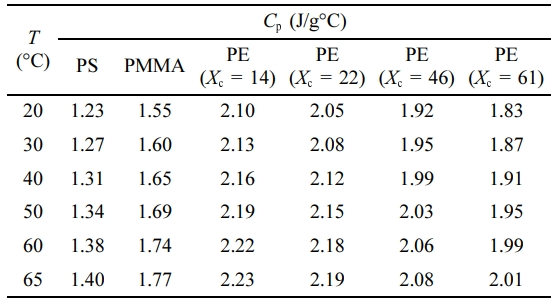experimental

Sheet samples of PE (4, 5, 10 mm thick), PS (5 mm thick) and PMMA (4, 5 and 10 mm thick) were used. Each sample was cut as a square with 30 cm side length. In order to change the degree of crystallinity of the PE samples, three samples were heated up to 180 oC for 2 h after inserting each one in a square stainless steel mold with 30 cm side length. The first sample was cooled at 3 oC/min rate, the second at 1 oC/min rate and the third sample at 0.5 oC/min rate, and then the degree of crystallinity was measured for the three samples using differential scanning calorimeter (DSC).16 DSC analysis have shown that the original sample of PE (before heating) has a degree of crystallinity of 14% (more amorphous). The degree of crystallinity is found to increase with decreasing the cooling rate. The degree of the crystallinity for the three PE samples (after heating and cooling process) is found to be 22%, 46%, and 61% for a cooling rate of 3, 1, and 0.5 oC/min, respectively.
The measurements of the thermal conductivity have carried out using a computerized thermal conductivity apparatus (Figure 1). This apparatus measures the thermal conductivity using heat flowmeter steady-state method.17 The specimen was sandwiched between two rubber sheets to remove the air gaps, and then it was placed between a hot plate and the heat flowmeter which is attached to a cold plate. The hot and cold plates are maintained at constant temperatures, the temperature of the two plates was measured using surface thermocouples. The apparatus is surrounded by insulating jacket to minimize the heat loss.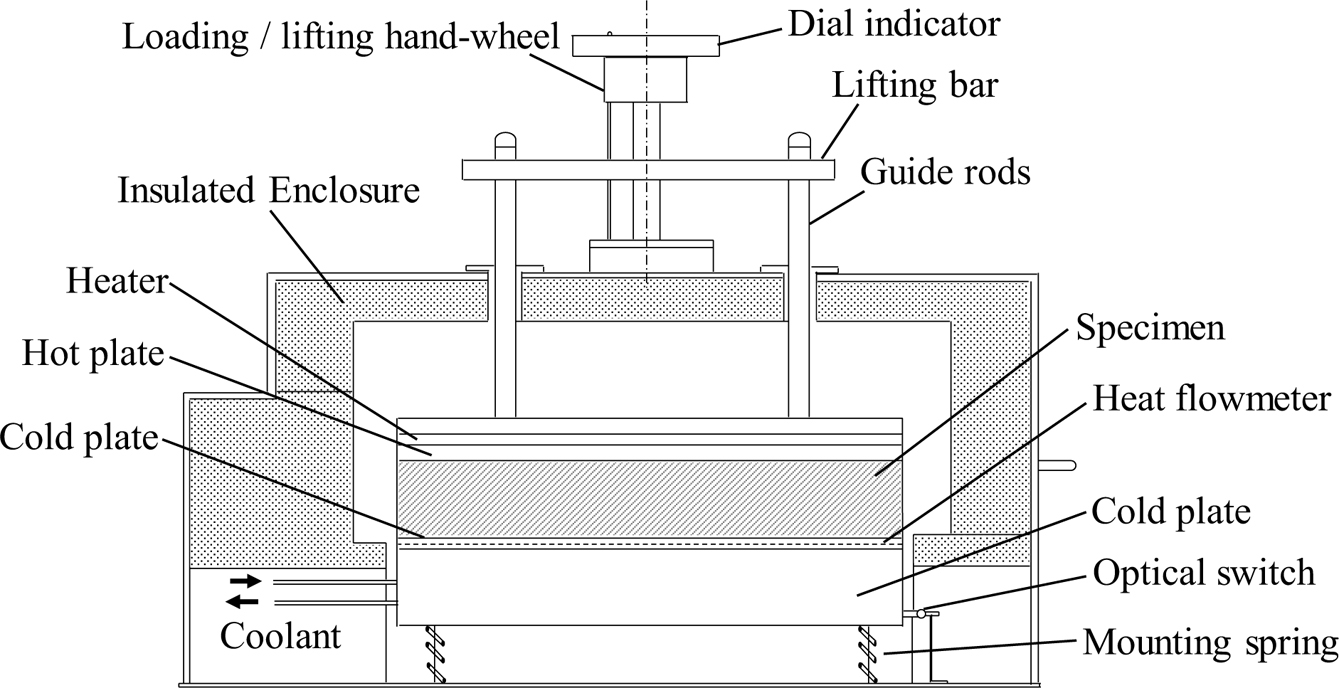Figure 1 Block diagram of the thermal conductivity apparatus
results and discussion

Polymer Structure Dependence. Thermal conductivity of PS, PMMA, and PE was measured at different temperatures: 20, 30, 40, 50, 60, and 65 oC. Figure 2 shows the temperature dependence of the thermal conductivity and thermal diffusivity of a 5 mm thick sample from each polymer. The thermal conductivity increases with temperature for the three polymers. For PS and PMMA (amorphous polymers) this increase is due to the increase in the specific heat with temperature in agreement with eq. (4). For PE (semicrystalline, 14% crystallinity), the increase in the thermal conductivity is due to larger percent of the amorphous content than the crystalline content in this sample, thus it behaves similar to amorphous polymers.18 The figure also shows that the thermal conductivity of PMMA is larger than that of PS, which is expected for amorphous polymers because the specific heat of PMMA is larger than that of PS.12 The thermal conductivity of PE is larger than that of the other two polymers because the PE is a semicrystalline polymer characterized by a more ordered structure, which in general yields less phonon boundary scattering and larger phonon mean free path, and thus higher thermal conductivity.19,20 The thermal diffusivity increases with temperature for the three polymers. Similar results have been reported recently on different polymeric materials.21,22 This could be explained through eq. (3), where the thermal conductivity increases with temperature, the volume density is almost temperature independent and the specific heat increases with temperature. Since the increase in the thermal conductivity is larger than the increase in the specific heat, the thermal diffusivity increases with temperature.
Specimen Thickness Dependence. In principle, thermal conductivity should be independent of specimen thickness or geometry. However, the measured thermal conductivity increases with increasing the specimen thickness for both amorphous and semicrystalline polymers as shown in Figure 3. This observation suggests a heat resistive interface (between the sample faces and the hot or cold plates) related to contact resistance. This contact resistance is essentially independent of the specimen thickness.23,24
The measured total thermal resistance of PMMA and PE polymers versus the sample thickness is shown in Figure 4. It is shown that the total thermal resistance increases linearly with the sample thickness. The total thermal resistance across the sample (the measured resistance) can be considered to be comprised of two parts, sample resistance (Rs) and contact resistance (Rc).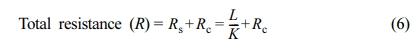where L is the sample thickness. This equation implies that a plot of the measured thermal resistance versus sample thickness should yield a straight line with slope (1/K) and intercept equals to the contact resistance.23,24 The calculated values of the contact resistance for PMMA and PE polymers from the intercepts of the lines in Figure 4 is listed in Table 2.
Degree of Crystallinty Dependence. The temperature dependence of the thermal conductivity was measured for four PE samples with different crystallinity (14, 22, 46, and 61%). As displayed in Figure 5, the thermal conductivity increases with increasing the degree of crystallinity. This is due to the higher ordered-structure in the samples with higher crystallinity; a case yields less boundary scattering and thus higher phonon mean free path.25,26 The results also show that the thermal conductivity for the first three samples (with crystallinity 14, 22 and 46%) increases with temperature, but for the fourth one (61% crystallinity) it decreases with temperature. This is because the amorphous content is larger than the crystalline one in the first three samples, so they behave similar to the amorphous polymers. In the fourth sample, the crystalline part is larger than the amorphous one, thus it behaves like a crystalline polymer and its thermal conductivity decreases with temperature.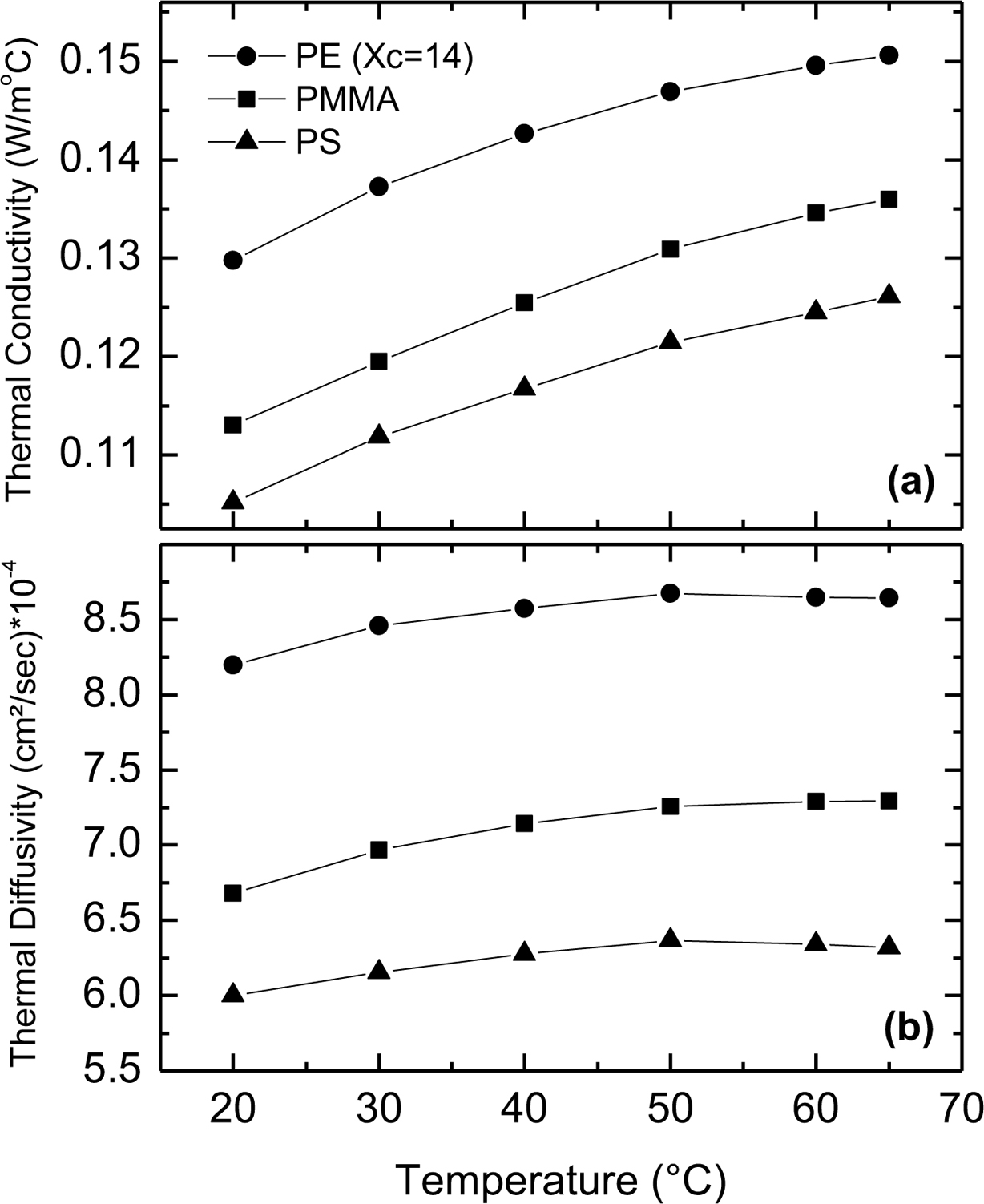Figure 2 Temperature dependence of (a) the thermal conductivity; (b) the thermal diffusivity of 5 mm thick samples of PS, PMMA and PE (Xc = 14%).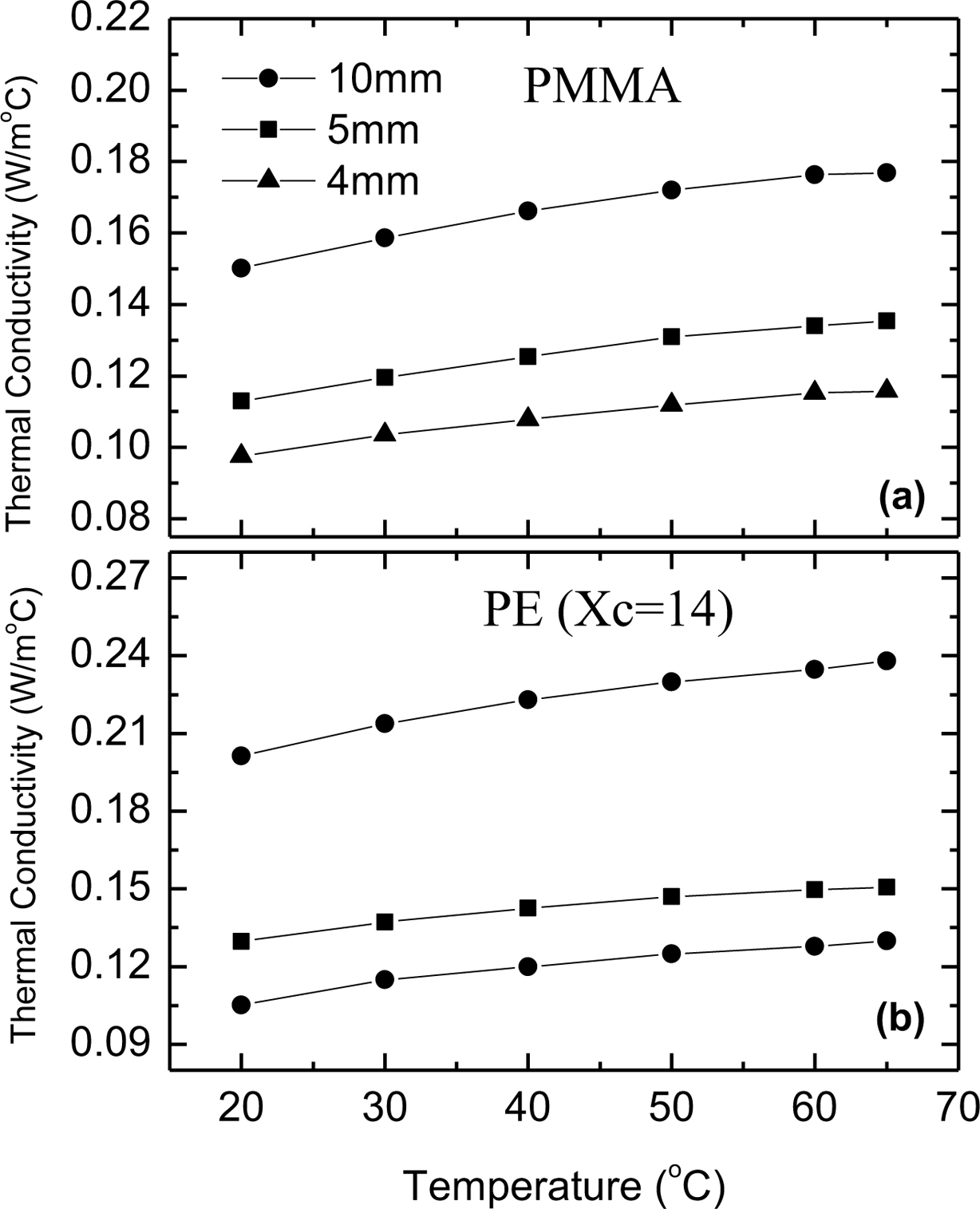Figure 3 Temperature dependence of the thermal conductivity of (a) PMMA; (b) PE (Xc = 14%) for different specimen thickness.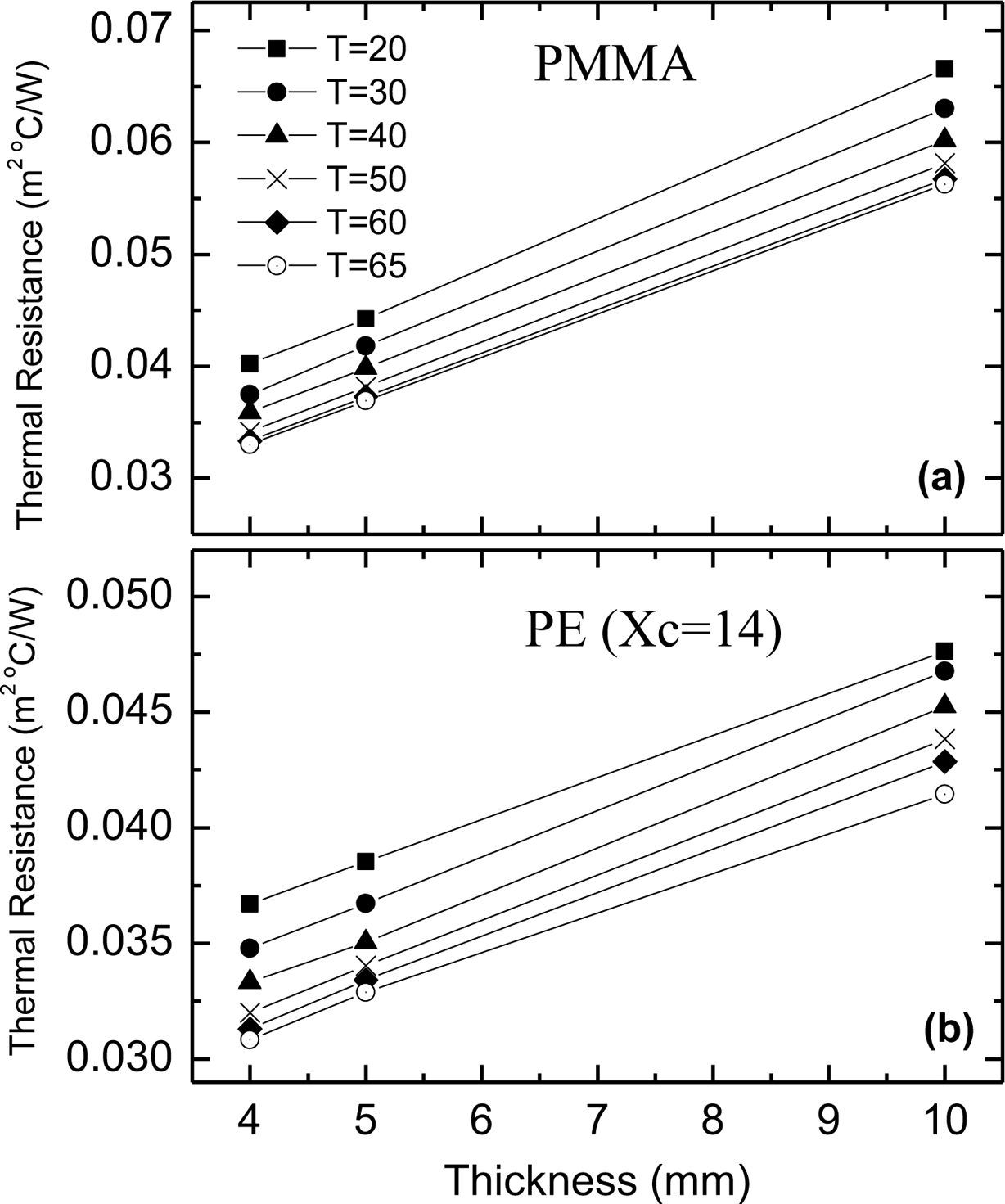Figure 4 Variation of thermal resistance with specimen thickness for (a) PMMA; (b) PE (Xc = 14%) samples.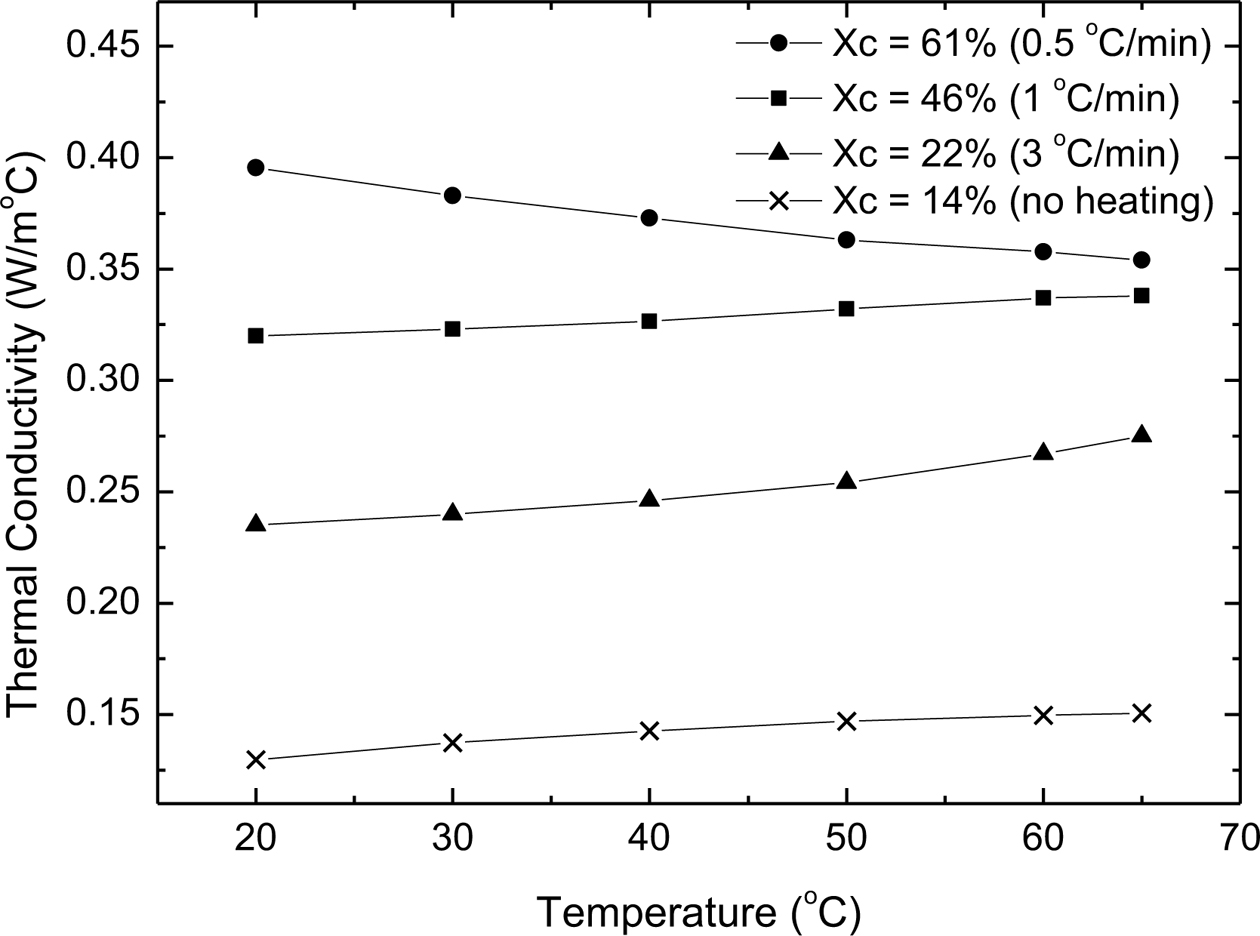Figure 5 Temperature dependence of the thermal conductivity of PE samples with different degrees of crystallinity. The cooling rate for each sample is written beside the degree of the crystallinity
 Table 2 Contact Resistance of PMMA and PE at Different Temperatures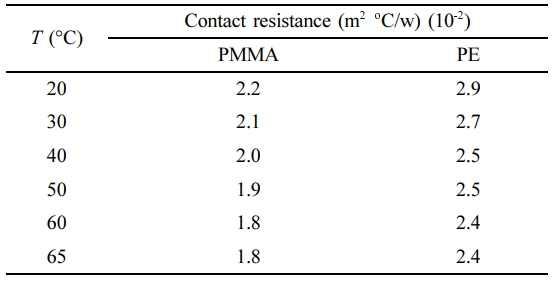conclusions

The thermal conductivity of PMMA, PS and PE polymers was studied as a function of temperature, degree of crystallinity and sample thickness. It was found that the thermal conductivity increases with temperature for amorphous and semicrystalline polymers with crystallinity less than 50%, and decreses with temperature for semicrystalline polymers with crystallinity greater than 50%. Moreover, the thermal conductivity of the crystalline polymers was found to be larger than that of the amorphous polymers. For semicrystalline polymers, the thermal conductivity increases by increasing the degree of crystallinity, and increasing the sample thickness leads to increasing the measured thermal conductivity due to the existence of the contact resistance.

References
• Polymer(Korea) 폴리머
• Frequency : Bimonthly(odd)
ISSN 0379-153X(Print)
ISSN 2234-8077(Online)
Abbr. Polym. Korea
• 2018 Impact Factor : 0.500
• Indexed in SCIE

###This Article

• 2021; 45(2): 281-285

Published online Mar 25, 2021

• 10.7317/pk.2021.45.2.281
• Received on Nov 2, 2020
• Revised on Nov 22, 2020
• Accepted on Dec 2, 2020

###Correspondence to

• B. Salameh
• Department of Applied Physics, Tafila Technical University, Tafila, Jordan

• E-mail: bsalameh@ttu.edu.jo
• ORCID:
0000-0002-2370-3484